Question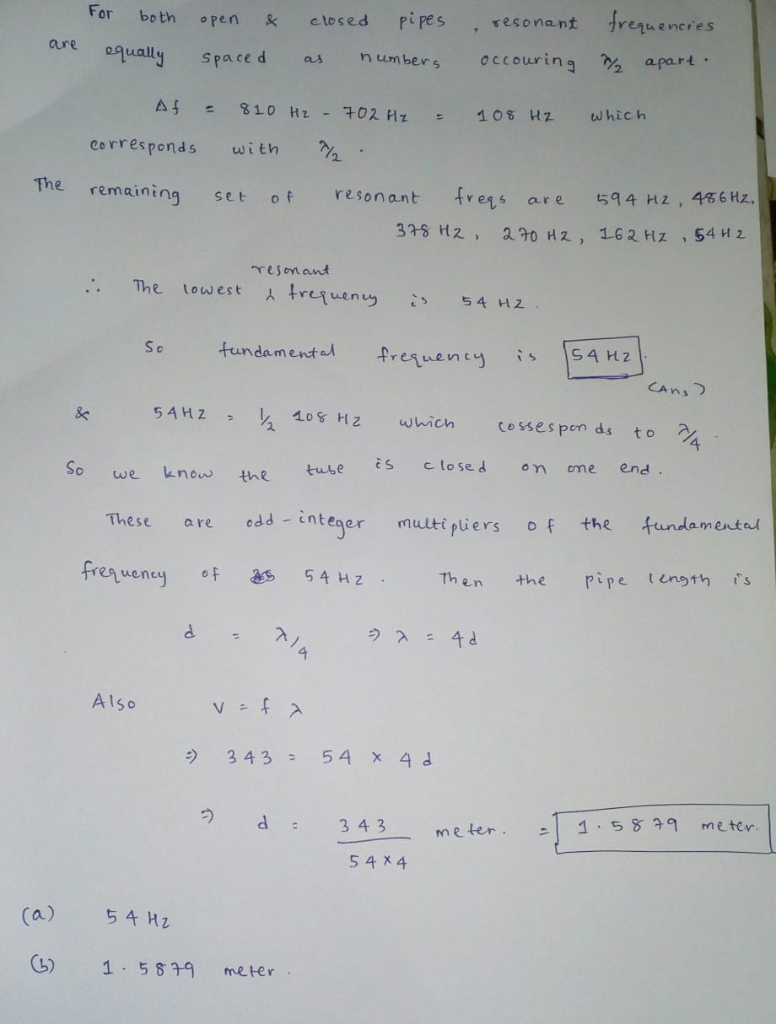please give an upvote if you like

#### Earn Coins

Coins can be redeemed for fabulous gifts.

Similar Homework Help Questions
• ### 6)Two adjacent natural frequencies of an organ pipe are determined to be 912 Hz and 1008...

6)Two adjacent natural frequencies of an organ pipe are determined to be 912 Hz and 1008 Hz. (Assume the speed of sound is 343 m/s.) (a) Calculate the fundamental frequency of this pipe. Answer must be in Hz (b) Calculate the length of this pipe. Answer must be in m 7)A train sounds its horn as it approaches an intersection. The horn can just be heard at a level of 60 dB by an observer 10 km away. (a) What...

• ### Organ pipe A with both ends open has a fundamental frequency of 320.0 Hz. The third...

Organ pipe A with both ends open has a fundamental frequency of 320.0 Hz. The third harmonic of organ pipe B with one end open has the same frequency as the second harmonic of pipe A. Assume a speed of sound of 343 m/s. What is the length of Pipe A? What is the length of Pipe B?

• ### An organ pipe is observed to produce 3 consecutive harmonics with frequencies of 189, 243 and...

An organ pipe is observed to produce 3 consecutive harmonics with frequencies of 189, 243 and 297 Hz. By how much does the tube need to be shortened so that the fundamental frequency is 60 Hz? The speed of sound is 343 m/s.

• ### Calculate the length of a pipe that has a fundamental frequency of 316 Hz. (Take the...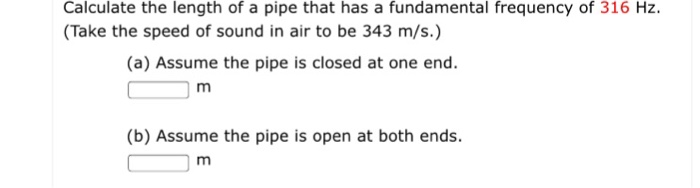Calculate the length of a pipe that has a fundamental frequency of 316 Hz. (Take the speed of sound in air to be 343 m/s.) Calculate the length of a pipe that has a fundamental frequency of 316 Hz. (Take the speed of sound in air to be 343 m/s.) (a) Assume the pipe is closed at one end (b) Assume the pipe is open at both ends

• ### Calculate the length of a pipe that has a fundamental frequency of 997 Hz. (Take the...

Calculate the length of a pipe that has a fundamental frequency of 997 Hz. (Take the speed of sound in air to be 343 m/s.) (a) Assume the pipe is closed at one end. m (b) Assume the pipe is open at both ends. m

• ### A certain pipe has resonant frequencies of 165 Hz, 275 Hz, and 385 Hz, with no...

A certain pipe has resonant frequencies of 165 Hz, 275 Hz, and 385 Hz, with no other resonant frequencies between these values. (a) Is this a pipe open at both ends or closed at one end? (b) What is the fundamental frequency of this pipe? (c) How long is this pipe? (Use the speed of sound in air at 20°C.)

• ### A pipe organ may contain tens of thousands of pipes of varying shapes, sizes and materials....

A pipe organ may contain tens of thousands of pipes of varying shapes, sizes and materials. (a) A pipe destined for an organ is open at both ends and has a length of 1.2 m. What is the wavelength of the longest standing wave that can be produced by this pipe? (b) The fundamental frequency produced by the pipe is measured to be 150 Hz. Calculate the speed of sound for the air in the pipe. (c) If one end...

• ### 18, + 03 points i Frenous Answars The shortest pipein a particular organ is 1.41 m (a) Determine the frequency (in Hz) of the ninth harmonic (at 0°C) if the pipe is closed at one end. 548.93 Racall t...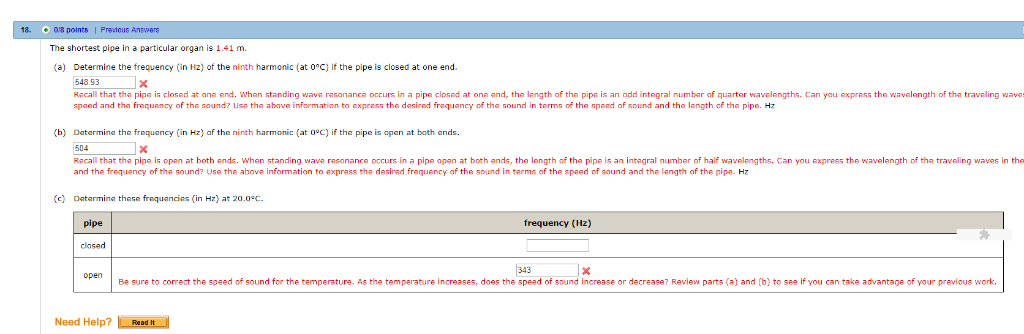18, + 03 points i Frenous Answars The shortest pipein a particular organ is 1.41 m (a) Determine the frequency (in Hz) of the ninth harmonic (at 0°C) if the pipe is closed at one end. 548.93 Racall that the pipe is closed at ane end. When standing wave rasonance occurs in a pipe closed at one and, the length of the pipe is an odd integral number of quarter wavelengths. Can you express the wavelength of the traveling wava...

• ### SOLUTION (A) Find the frequencies if the pipe is open at both ends. _V 343 m/s...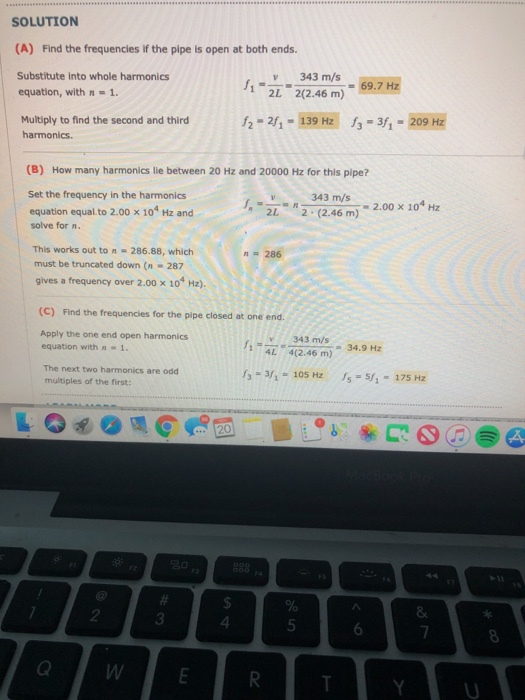SOLUTION (A) Find the frequencies if the pipe is open at both ends. _V 343 m/s Substitute into whole harmonics equation, with n = 1. 11-222(2.46 m) = 69.7 Hz Multiply to find the second and third harmonics. 12 - 27 - 139 Hz 13 = 3f7 - 209 Hz (B) How many harmonics lle between 20 Hz and 20000 Hz for this pipe? 343 m/s Set the frequency in the harmonics equation equal to 2.00 x 104 Hz and...

• ### An organ pipe is 2.0-m long. Assume the pipe is cylindrical with one closd and one...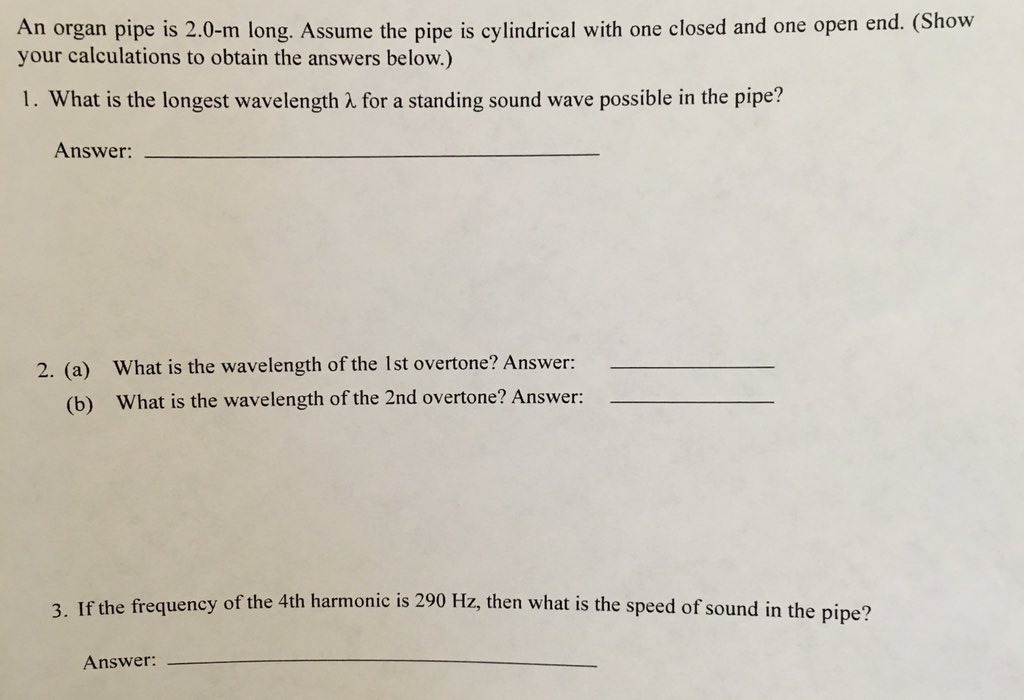An organ pipe is 2.0-m long. Assume the pipe is cylindrical with one closd and one open end. 1) What is the longest wavelength for a standing sound wave possible in the pipe? 2) What is the wavelength of the first 1st overtone? What is the wavelength of the 2nd overtone? 3) If the frequency of the 4th harmonic is 290 hz, what is the speed of sound in the pipe? An organ pipe is 2.0-m long. Assume the pipe...